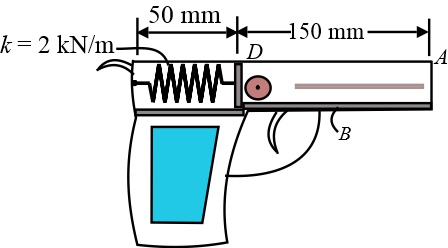# The spring in the toy gun has an unstretched length of 100 mm. It is compressed and locked in the...

## Question:

The spring in the toy gun has an unstretched length of 100 mm. It is compressed and locked in the position shown. In this position, the spring is exerting a force of 100 N on the ball. When the trigger is pulled, the spring unstretches 12.5 mm, and the 30-g ball moves along the barrel.

Determine the initial acceleration of the ball the moment the trigger is pulled. Neglect friction.## Spring-Mass System:

We consider a body with mass M is attached to a spring with spring constant k. Let a force F acts on the body make the spring compress by x.

So the restoring force in the spring is F = k.x.

## Answer and Explanation: 1

Become a Study.com member to unlock this answer! Create your account

Given:

• Unstretched length of the spring is L = 100 mm.
• Length of the spring after compression is l = 50 mm.
• Mass of the ball is m = 30 g =0.03 kg.
• Sp...

See full answer below.

#### Learn more about this topic:Practice Applying Spring Constant Formulas

from

Chapter 17 / Lesson 11
3.4K

In this lesson, you'll have the chance to practice using the spring constant formula. The lesson includes four problems of medium difficulty involving a variety of real-life applications.